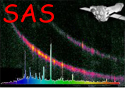XMM-Newton Science Analysis System

arfgen (arfgen-1.104) [xmmsas_20230412_1735-21.0.0]

## Encircled energy correction

In the case of a point source, arfgen can correct for the flux that is outside the region(s) over which the spectrum was accumulated. These regions are read from the spectrum's DSS information. The correction works by taking a model of the PSF from the CAL, centred at the source position and calculating the total fraction of that PSF within the regions of interest.

To enable the encircled energy correction for point sources, the parameter extendedsource must be set to false, as well as modelee being set to true.

The encircled energy correction is energy dependent, so ideally the EE should be computed for each and every point on the energy grid. However, given that the EE calculation is computationally intensive, the option to resample the energy grid is provided by the parameter eegridfactor. The resultant EE correction vector is interpolated back onto the original energy grid, and each ARF element is corrected using the corresponding EE correction factor. See section 10 for further information.

By default arfgen uses a fomulation of the PSF as a function of energy and off-axis angle, known as the ELLBETA model, to calculate the encircled energy correction. This model is valid for all extraction region shapes as it works by summing up contributions to the PSF over a grid of pixels which fall within the region. The resolution of this grid (and of the bad pixel calculation) is set by the parameter badpixelresolution which has a default of 0.5 arcseconds. The PSF model may be overridden using the psfmodel parameter.

XMM-Newton SOC -- 2023-04-16